## TEI-L@LISTSERV.BROWN.EDU

#### View:

 Message:[First|Previous|Next|Last] By Topic:[First|Previous|Next|Last] By Author:[First|Previous|Next|Last] Font: Proportional Font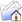LISTSERV Archives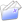TEI-L HomeTEI-L November 1991

Subject:Encoding schemes for graphs, directed graphs and trees

From:Terry Langendoen <[log in to unmask]>

Reply-To:Terry Langendoen <[log in to unmask]>

Date:Tue, 26 Nov 1991 19:58:09 MST

Content-Type:text/plain

Parts/Attachments: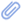text/plain (297 lines)
 ``` Encoding of graphs, digraphs and trees authorD. Terence Langendoen Preliminary version. This material is based on Gary Chartrand and Linda Lesniak, Graphs and digraphs, second ed., Monterey, CA: Wadsworth Brooks/Cole Advanced Books and Software, 1986. DTL 24 November 1991 began first draft 0 DTL 25-26 November 1991 revised and extended tree material 1 Steven Zepp 26 November, 1991 fixed header and typos; checked that document and dtd parses 2

This material is based on Gary Chartrand and Linda Lesniak, Graphs and digraphs, second ed., Monterey, CA: Wadsworth and Brooks/Cole Advanced Books and Software, 1986. Graphs What graphs are

A graph is a collection of vertices together with a collection of pairs of those vertices, called edges. Each vertex in an edge is said to be incident with that edge, and the two vertices which make up an edge are said to be adjacent. In the encoding scheme developed here, vertex is used to indicate the vertices. Associated with each vertex (i.e., enclosed within each vertex) are pointers to the vertices which are adjacent to that vertex; each pointer is represented by an empty adjac. In a fully specified encoding of a graph, each edge occurs twice, once for each vertex that is incident with it. A graph is an appropriate data structure for representing linkages among data points, the points corresponding to the vertices and the linkages corresponding to the edges. Partial DTD for graphs

<![ CDATA [ <!ELEMENT graph - - (vertex)+ > <!ATTLIST graph %global.attributes;                 order NUMBER #IMPLIED                 size NUMBER #IMPLIED > <!ELEMENT vertex - o (fs?, (adjac)*) > <!ATTLIST vertex %global.attributes;                  degree NUMBER #IMPLIED > <!ELEMENT adjac - o EMPTY > <!ATTLIST adjac %global.attributes;                 target IDREF #REQUIRED > ]] Sample graph to be encoded

In this sketch, the edges are represented by dashed lines, and the + sign, for indicating a 90 degree bend (which is not significant, and is used simply to overcome the inability to draw diagonal lines).

<![ CDATA [           +----u----+           | |           v---------w-----x y ]] Encoding of sample graph <![ CDATA [ <graph n=1 id=g1 order=5 size=4> <vertex n=u id=g1u degree=2>   <adjac target=g1v>   <adjac target=g1w> <vertex n=v id=g1v degree=2>   <adjac target=g1u>   <adjac target=g1w> <vertex n=w id=g1w degree=3>   <adjac target=g1u>   <adjac target=g1v>   <adjac target=g1x> <vertex n=x id=g1x degree=1>   <adjac target=g1w> <vertex n=y id=g1y degree=0> </graph> ]] Digraphs What digraphs are

A digraph or directed graph differs from a graph in that the linkage between the vertices is ordered. Each linkage is called an arc. We also rename vertex as node, since the corresponding tag has a different content model and associated attributes. In the illustrative diagram, the ordering is indicated by the symbols > and <. Partial DTD for digraphs

><![ CDATA [ v<!ELEMENT digraph - - (node)+ > <!ATTLIST digraph %global.attributes;                   order NUMBER #IMPLIED                   size NUMBER #IMPLIED > <!ELEMENT node - o (fs?, (adjac.to* & adjac.from*)) > <!ATTLIST node %global.attributes;                in.degree NUMBER #IMPLIED                out.degree NUMBER #IMPLIED > <!ELEMENT (adjac.to, adjac.from) - o EMPTY > <!ATTLIST (adjac.to, adjac.from)                      %global.attributes;                      target IDREF #REQUIRED > ]] Sample digraph to be encoded <![ CDATA [           +-<--u--<-+           | |           v---->----w---->----x y                     | |                     +----<----+ ]] Encoding of sample digraph <![ CDATA [ <digraph n=1 id=d1 order=5 size=5> <node n=u id=d1u in.degree=1 out.degree=1>   <adjac.to target=d1v>   <adjac.from target=d1w> <node n=v id=d1v in.degree=1 out.degree=1>   <adjac.from target=d1u>   <adjac.to target=d1w> <node n=w id=d1w in.degree=2 out.degree=2>   <adjac.to target=d1u>   <adjac.to target=d1x>   <adjac.from target=d1v>   <adjac.from target=d1x> <node n=x id=d1x in.degree=1 out.degree=1>   <adjac.to target=d1w>   <adjac.from target=d1w> <node n=y id=d1y in.degree=0 out.degree=0> </digraph> ]] Trees What trees are

A tree is a connected acyclic graph. That is, it is possible to follow a path from any vertex to any other vertex, but there are no loops. A rooted tree is a digraph based on a tree; that is, the arcs in the digraph correspond to the edges of a tree such that there is exactly one node (called the root) for which there is a path (using the arcs) from that node to all other nodes. For TEI purposes, we may ignore all trees except for rooted trees, and hence we shall use tree for rooted trees. A node adjacent to a given node is called a child of that node, and the node adjacent from a given node is called its parent. A node with both a parent and children is called an internal node, for which we use i.node. A node with no children is called a leaf. If the children of a node are ordered, then we say that that node is ordered. If all the nodes of a tree are ordered, then we say that the tree is an ordered tree. If some of the nodes of a tree are ordered and others are not, then the tree is a partially ordered tree. The ordering of nodes and trees may be specified by an attribute. We assume that trees are ordered by default. Finally, we permit nodes to be specified as following other nodes, which (under normal ordering circumstances) they would be assumed to precede, giving rise to crossing arcs. An example is given below.

In TEI AI1 W10 a DTD for a different formalization of trees is given. The tree there should be renamed h.tree or some such. The shrub should also be retained for its usefulness for special purposes, incorporating a change suggested by Gary Simons at the Myrdal meeting. Partial DTD for trees

<![ CDATA [ <!ELEMENT tree - - (root & (leaf+ & i.node*)*) > <!ATTLIST tree %global.attributes;                ordered (yes | no) yes                order NUMBER #IMPLIED                size NUMBER #IMPLIED > <!ELEMENT root - o (fs?, (child)*) > <!ELEMENT i.node - o (fs?, (child)+) > <!ATTLIST root %global.attributes;                ordered (yes | no) yes                out.degree NUMBER #IMPLIED > <!ATTLIST i.node %global.attributes;                  ordered (yes | no) yes                  out.degree NUMBER #IMPLIED                  parent IDREF #IMPLIED                  follow IDREF #IMPLIED > <!ELEMENT leaf - o (fs)? > <!ATTLIST leaf %global.attributes;                parent IDREF #IMPLIED                follow IDREF #IMPLIED > <!ELEMENT child - o EMPTY > <!ATTLIST child %global.attributes;                 target IDREF #REQUIRED > ]] First sample tree to be encoded

In this sketch, we leave out the arrows indicating directionality, on the assumption that the root is at the top, and that the direction of the arcs is from root to leaf. We also assume that this tree is partially ordered, with the internal node v ordered and the root node u unordered (indicated by double broken lines).

<![ CDATA [             +====u====+           | |      +----v----+ w      | | |      V V V      x y z   ]] Encoding of first sample tree <![ CDATA [ <tree n=1 id=t1 ordered=no order=6 size=5> <leaf n=x id=t1x parent=t1v> <leaf n=y id=t1y parent=t1v> <leaf n=z id=t1z parent=t1v> <i.node n=v id=t1v parent=t1u ordered=yes out.degree=3>   <child target=t1x>   <child target=t1y>   <child target=t1z> <leaf n=w id=t1w parent=t1u> <root n=u id=t1u out.degree=2>   <child target=t1v>   <child target=t1w> </tree> ]] Second sample tree to be encoded

In this illustration, we assume that the tree is ordered and that the node PT follows the node PN.

<![ CDATA [             +----VP---+           | |           | +----x----+           | | | |           | | PN |      +----VB---+ | PT     VB | |      | | |    look them up   ]] Encoding of second sample tree <![ CDATA [ <tree n=2 id=t2 order=8 size=7> <leaf n=look id=t2look parent=t2VB2> <leaf n=them id=t2them parent=t2PN> <leaf n=up id=t2up parent=t2PT> <i.node n=VB id=t2VB2 parent=t2VB1 out.degree=1>   <child target=t2look> <i.node n=PN id=t2PN parent=t2VP out.degree=1>   <child target=t2them> <i.node n=PT id=t2PT parent=t2VB1 follow=t2PN out.degree=1>   <child target=t2up> <i.node n=VB id=t2VB1 parent=t2VP out.degree=2>   <child target=t2VB2>   <child target=t2PT> <root n=VP id=t2VP out.degree=2>   <child target=t2VB1>   <child target=t2PN> </tree>
```

Advanced Options

#### Options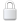Log In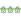Get Password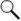Search Archives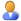Subscribe or Unsubscribe